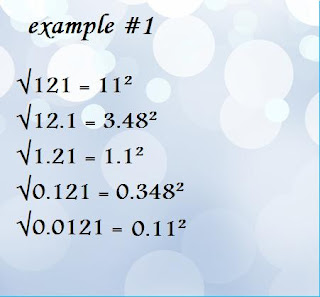Monday, November 19, 2012

Math Notes- November 19,2012

To determine perfect squares and surds.I noticed that the surds have an odd number of decimals. (Notice the red line on the picture above). If it is a perfect square, it would have an even amount of decimals.
for example:
√0.0081 = 0.09²

There is an even amount of decimals (in this case, there are

four) which tells me that it's square root is not a surd.

SQUARE ROOT OF FRACTIONS: